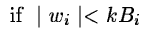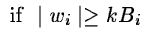Next: Hierarchical adaptive filtering Up: Noise reduction from the Previous: Hierarchical Wiener filtering

## Adaptive filtering from the wavelet transform

In the preceding algorithm we have assumed the properties of the signal and the noise to be stationary. The wavelet transform was first used to obtain an algorithm which is faster than classical Wiener Filtering. Then we took into account the correlation between two different scales. In this way we got a filtering with stationary properties. In fact, these hypotheses were too simple, because in general the signal may not arise from a Gaussian stochastic process. Knowing the noise distribution, we can determine the statistically significant level at each scale of the measured wavelet coefficients. If wi(x) is very weak, this level is not significant and could be due to noise. Then the hypothesis that the value Wi(x) is null is not forbidden. In the opposite case where wi(x) is significant, we keep its value. If the noise is Gaussian, we write:

 Wi = 0(14.90) Wi = wi(14.91)

Generally, we choose k = 3.

With a filter bank we have a biunivocity between the image and its transform, so that the thresholded transform leads to only one restored image. Some experiments show us that uncontrolled artifacts appear for high level thresholding (k=3). The decimation done at each step on the wavelet transform takes into account the knowledge of the coefficients at further resolutions. The thresholding sets to zero the intrinsic small terms which play their part in the reconstruction. With the lattice filter the situation is very different. No decimation is done and the thresholding keeps all significant coefficients. Where the coefficients are set to zero, we do not put zero, but we say that these values are unknown. The redundancy is used to restore them. Before the thresholding we have a redundant transform, which can be decimated, after the thresholding we get a set of coefficients from which we wish to restore in image.

If one applies the reconstruction algorithm, then it is not guaranteed that the wavelet transform of the restored image will give the same values for the coefficients. This is not important in the case where they are not significant, but otherwise the same values must be found. If W(s)i are the coefficients obtained by the thresholding, then we require Wi(x) such that:

 P.Wi(x) = W(s)i(x) (14.92)

where P is the non linear operator which performs the inverse transform, the wavelet transform, and the thresholding. An alternative is to use the following iterative solution which is similar to Van Cittert's algorithm:
 W(n)i(x) = Wi(s)(x) + Wi(n-1)(x) - P.Wi(n-1)(x) (14.93)

for the significant coefficients () and:
 Wi(n)(x) = Wi(n-1)(x) (14.94)

for the non significant coefficients ( Wi(s)(x) = 0).

The algorithm is the following one:

1.
Compute the wavelet transform of the data. We get wi.
2.
Estimate the standard deviation of the noise B0 of the first plane from the histogram of w0.
3.
Estimate the standard deviation of the noise Bi from B0 at each scale.
4.
Estimate the significant level at each scale, and threshold.
5.
Initialize: W(0)i(x) = Wi(s)(x)
6.
Reconstruct the picture by using the iterative method.

The thresholding may introduce negative values in the resulting image. A positivity constraint can be introduced in the iterative process, by thresholding the restored image. The algorithm converges after five or six iterations.Next: Hierarchical adaptive filtering Up: Noise reduction from the Previous: Hierarchical Wiener filtering
Petra Nass
1999-06-15